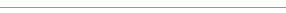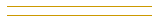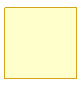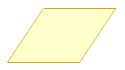Name: Julia Grade: 3 My perimeter is 12 cm. My sides are all equal. The sum of half my sides is equal to 6 cm. How many sides do I have? What is my name? Please help Thanks Hi Julia, I imagine that I am trying to make this shape. The perimeter is 12 cm, so I imagine that I have a 12 cm piece of string.Since "The sum of half my sides is equal to 6 cm.", cut the string in half get two pieces, each 6 cm long.Since two sides are not enough to make a shape and "My sides are all equal.", cut each piece in half again to get 4 pieces of length 3cm each. Put the 4 pieces together to form a shape. Do you know what it is called?I suppose that you could put the pieces together like this.Do you know what this is called? You could even cut each of the two 6 cm pieces into 3, to get 6 pieces of length 2 cm, and then form a shape. I like this question because there are lots of correct answers. Cheers, Penny Go to Math Central